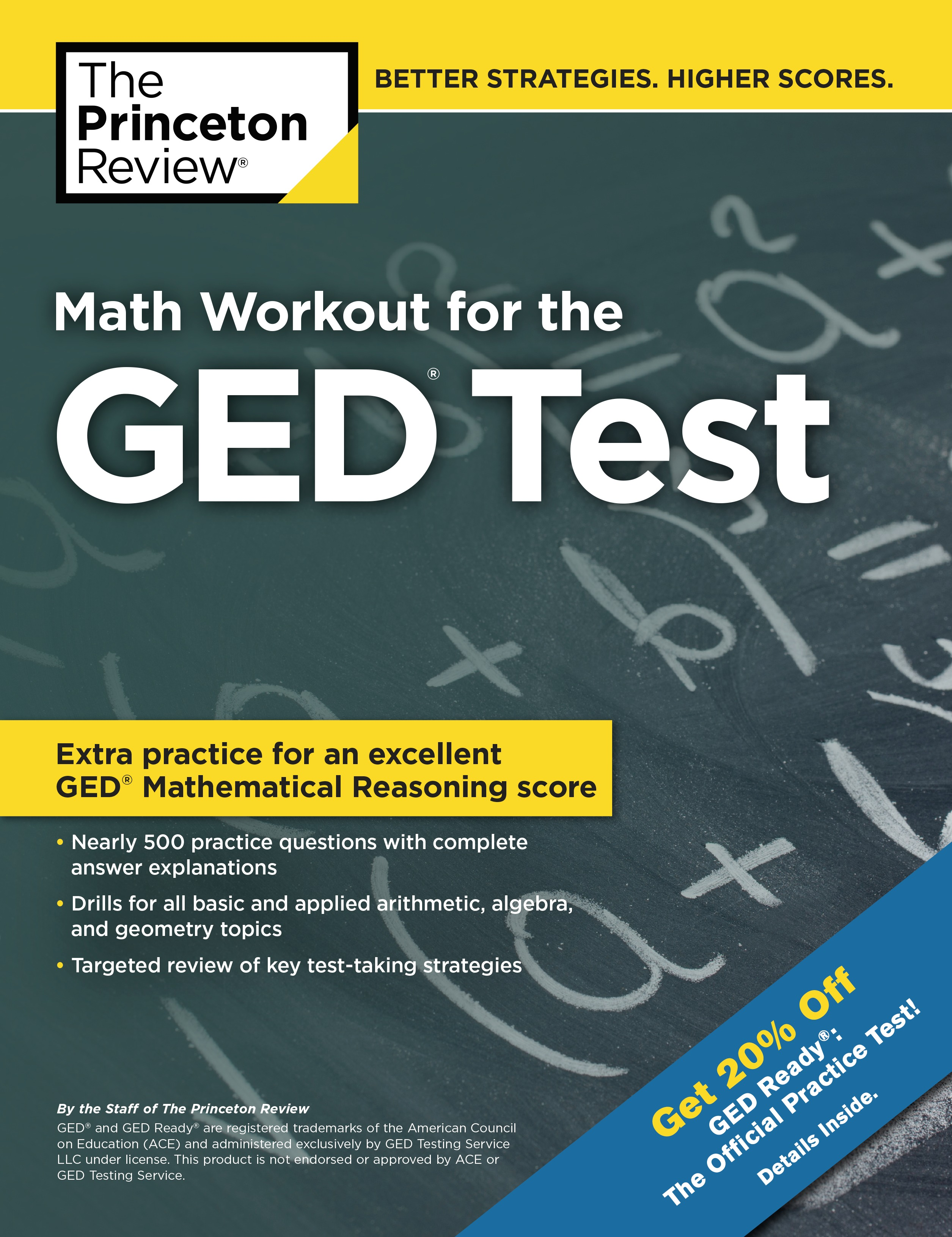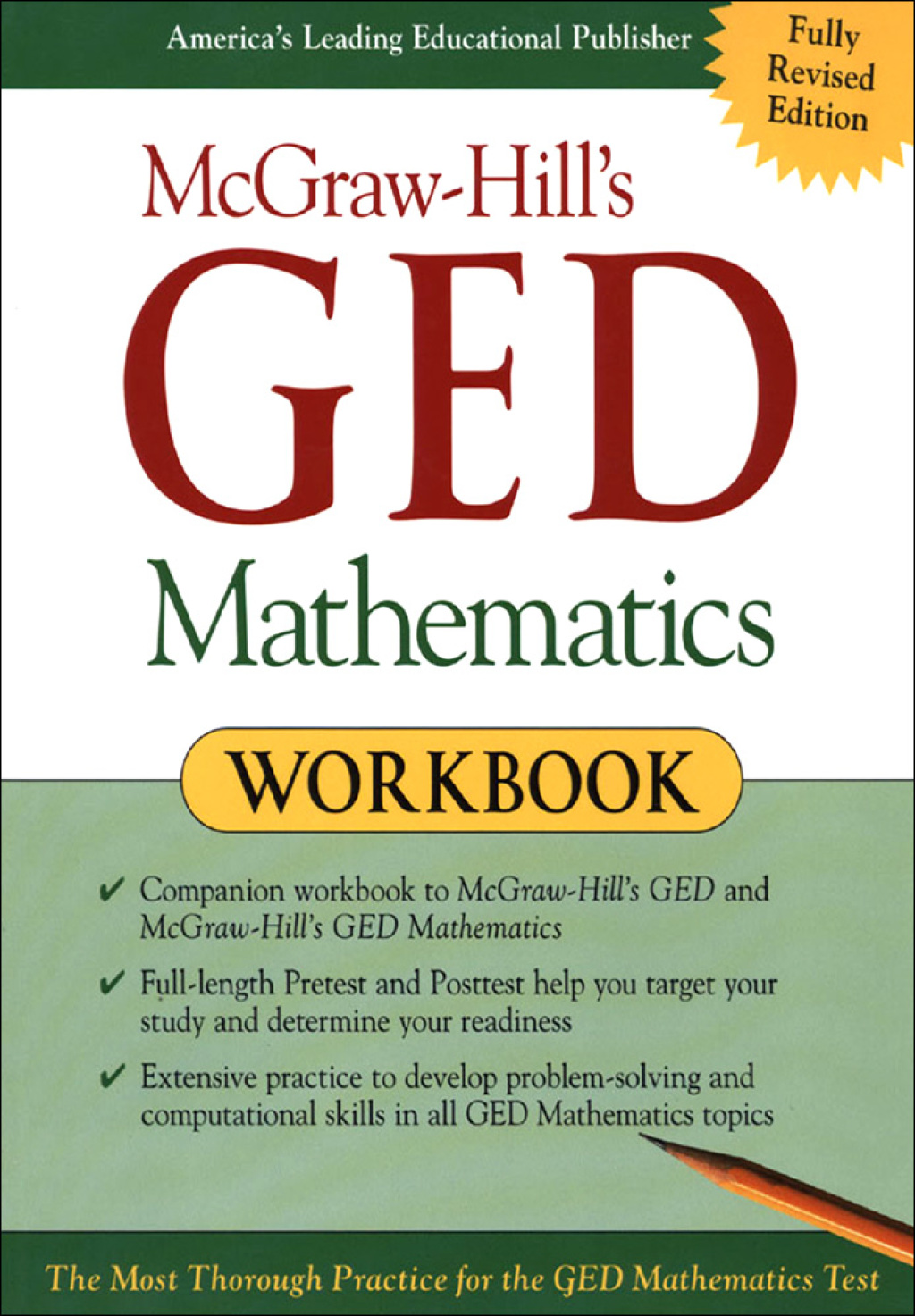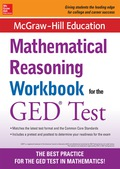GED MathHome  Algebra  Geometry  Arithmetic  Trigonometry  Probability/Statistics  Calculus  Mechanics GED  SAT  ACT  College Mathematics  GRE  School Supplies  About Us

GED Math

The GED Math Content
 Number Operations (Arithmetic) Percent, Ratio and ProportionsOrder of Operations, Metric Units Simple Interest, Properties of Real Numbers Integers, Natural NumbersOperations with Signed NumbersRational and Irrational NumbersPrime Numbers, Composite NumbersGreatest Common Divisor (GCD)Least Common Multiple (LCM)Absolute Value, Fractions, DecimalsAssociative, Distributive and Commutative Properties, Sequence of NumbersScientific Notation, Distance, Speed, Time. Measurement and Geometry The Right Angle, Acute, Obtuse Straight and Reflex AnglesComplementary and Supplementary AnglesVertically Opposite AnglesAlternate and Corresponding AnglesAreas and Perimeter of a Square, Rectangle and Triangle, Areas and Circumference of a CircleAreas of Polygons, Trapezoid and ParallelogramVolumes of Prisms, a Right Circular Cylinder, Sphere, Pyramid and Cone.Co-ordinates and The Straight LineLength of a Straight Line Slope of a Straight LineGraphs of a Straight Line Equation of a Straight Line. Algebra, Functions and Patterns  Laws of ExponentsQuadratic Solutions and GraphsPythagorean Theorem, Simple Equations Data Analysis, Statistics and Probability Mean, Median, Mode, Probability, Frequency

 Electronic Math BooksPaperback Math Books (New and Used)Example Problems
Number Operations
 Distance, Speed, Time 1. A trucker drove for 4 hours at an average speed of 60 miles per hour (mph) and then for 3 hours at an average speed of 30 mph. What was his total distance traveled? There are two parts to his travel. Applying the formula: Distance = Speed X Time and substituting the values,                      First part, Distance = 60  X  4 = 240 miles               Second part, Distance = 30  X  3 = 90 miles                               Total Distance traveled = 240 + 90                                                          = 330 miles Fractions  3. Solve the following: (i) 2⅔  X  3�      (ii) 5�  +  2�        (iii) 1⅝  �  ⅜                                                  2⅔  X  3�           5�  +  2�                1⅝  �  ⅜                                                 8/3 X 7/2               � + � = 1             13/8  X  8/3                                                    = 56/6         and 5 + 2 = 7                    = 13/3                                        = 9⅓              7 + 1 = 8                   = 4⅓ Percent  2. The computer I need to buy costs \$1,200.00. So far I have managed to save only 65% of the  cost. How much more money will I need to make the purchase?                      Percent means out of 100. Therefore 65% is 65/100                           Money save so far is 65/100  X  1200   =   65 X 1200                                                                                                            100                                                                                                       =  780                                                                                    That is \$780.00 If the computer costs \$1,200.00 and I have only \$780.00, I will need then                                          \$1,200.00 - \$780.00   =   \$420.00 Percent  4. (i) What is 14% of 250?  (ii) 40% of what number is 30? (iii) 80 is what percent of 200?        (i)  14% is 14/100                    (ii)  Let the number be T                  (iii)  80/200) X 100                 (14/100) X 250                          40/100 of T = 30                              (8/20) X 100 = 800/20                           = 35                                   40T = 30 X 100                                = 40%                                                              T = (30 X 100) � 40                                                                                      = 3000 � 40                                                                 = 75

Algebra
 Simple Equations  Solve the equations:       (i) -3x = 12       (ii) 4x + 2x = 66            (iii) 5a + 3a = 120             (iv) Solve for x in: 6(x - 3) + x = 24            -3x = 12              4x + 2x = 66                   5a + 3a = 120                                               6(x - 3) + x = 24               x = 12/-3                  6x = 66                            8a = 120                                               6x - 18 + x = 24              x = -4                     x = 66/6                            a = 120/8                                       7x = 24 + 18 = 42                                        x = 11                      a = 15                                       x = 42/7 = 6                                                                                                                            x = 6

Measurement and Geometry
 Area of a Circle 1. Find the area of a circle with radius 3.5 cm.                Area of the circle = πr� where π = 3.14                Substituting the values:                                            Area = 3.14 X (3.5)�                                                     = 3.14 X 12.25 12.25                                                    = 38.465 sq. cm. Circumference of a Circle   2. What is the circumference of the same circle described above?         Circumference of the circle = π X diameter.            If the radius of the circle is 3.5 cm then its diameter is 2 X 3.5 = 7 cm.                                                       Circumference therefore is 3.14 X 7 = 21.98 cm

 Perimeter of a Square  3. If one side of a square is 4 cm, what is the perimeter of the square?            Perimeter of the square is the total distance around  = 4 X 4 cm or 4 + 4 + 4 + 4                                                                                                        = 16 cmfor Math Practice, GED      SAT   ACT   College Math   GRE

 More School Supplies and other useful items *  * * * *  * Household Supplies